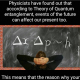# Calculating the Pair Correlation Function in Python

The pair correlation function, also known as the radial distribution function, is a way to characterize the distribution of particles on a two-dimensional plane or in a three-dimensional space. Please check out Eric Weeks’ web site for an introduction to pair correlation functions. He has written some routines in IDL to compute these functions. Using his foundation, I have written some simple routines in Python to compute 2D and 3D pair correlation functions.

The pair correlation code is available in the Shocksolution_Examples repository on GitHub. If you are familiar with Git, you can clone the entire repository, or simply right-click and save the individual files that you need.

These books are helpful if you’re working with NumPy:### Two-Dimensional Pair Correlation (Radial Distribution) Function

The pair correlation function g(r) is a measure of the structure that is present in a collection of particles. To validate the code, and understand how it works, I generated some populations of particles and plotted the pair correlation functions. The populations were generated by random sequential adsorption (RSA) in which each particle was added to the population in a random position. If the position caused the particle to overlap an existing one, the position was rejected and a new one was generated. The first plot shows a representative low-density population of particles. The red particles were used to compute g(r) because a circle of radius rMax centered at those particles lies entirely within the domain. Because the populations were randomly generated, the plotted g(r) is averaged over fifty different populations (dotted lines are +/- one standard deviation). Note that g(r) is never actually negative!

The pair correlation function is almost uniform for this distribution of particles. Because the particles are randomly located and not tightly packed, they have essentially no structure. g(r) is zero for r<1 because the particles have radius one and they are not allowed to overlap.

Now we will test the function on randomly distributed particles that are more densely packed.

The pair correlation function now shows some structure for distances close to the reference particle. Although the particles are still randomly distributed, the higher density forces them to take on some short-range structure as they try to fit in the square without overlapping.

Now we will examine the pair correlation function of a highly ordered array of particles. These particles are hexagonally packed, which is mathematically proven to be the highest-density arrangement for circles on a plane.

The pair correlation function now reflects the large amount of order in the particles. If you draw a circle surrounded by hexagonally packed circles and do a little geometry, you can see that each “spike” corresponds to the center-center distance between the reference circle and another circle.

### Three-Dimensional Pair Correlation (Radial Distribution) Function

#### Validation

The 3D pair correlation function was validated using a low-density and high-density distribution of particles within a cube. Because packing spheres in 3D space is much more involved than 2D circle packing, I did not test the routine on packed spheres. If you do, I’d like to hear about it.

Now we will look at spherical particles which are more densely packed.

Short-range order is evident in the pair correlation function.

### 9 thoughts on “Calculating the Pair Correlation Function in Python”

1.Hi sir! please guide me how i calculate the average number of neighboring particle of two different particles in phase separation and radius of gyration (distribution function) for each particles

2.That’s a pretty complicated question, so I can’t answer it here. Please email me at the address shown above if you want to hire me to work on it.

3.Good job! Keep on!

4.Thanks, this has been very helpful! 🙂

5.Arunjyoti Sinha Roy

Thanks a lot, it has been of huge help.

6.Thank you for the script! I went through the python script to learn how RDF is calculated.
I have a question: I realised that your RDF script draws edges centred around 0,0,0 cartesian coordinate. I am trying to calculate/plot RDF of a nanocluster and the centre of the mass is not 0,0,0.
Could you please give me advice how should I calculate RDF for a nanocluster?
The .xyz file is :
16
Energy -200.0
A 1.977502779 1.825612486 -1.078815994
A 0.073484389 -2.915354734 -1.169129839
A -1.682844787 -1.543503043 -2.245494959
A 0.226989000 0.103121000 -0.417822000
B -0.693058883 0.156772052 1.151824239
B -1.448474661 -3.517890885 -2.298992143
B -1.458396055 -1.997135497 -0.344566446
B 0.985126104 -4.427396897 -0.775735938
B -3.121800014 -1.219516661 -3.292662828
B 2.017385825 0.679529254 0.430803534
B 0.212637914 -1.726148783 -2.725852021
B 3.217540502 3.061671270 -1.526834132
B 1.456477430 0.338098844 -2.124519369
B -1.187423538 0.191670365 -1.675742064
B 0.143729055 2.143022931 -0.717464213
B 1.083320805 -1.581985916 -0.284118283

1.The coordinate system can be set up arbitrarily to meet the needs of your calculation. You can find the actual center of mass of your particles and then translate all particles so that your coordinate system is centered at (0,0).

7.Thanks for the script! Should I modify the code for periodic boundary condition only in one direction (y-direction)?

This site uses Akismet to reduce spam. Learn how your comment data is processed.